Author: Oscar Cronquist Article last updated on May 11, 2022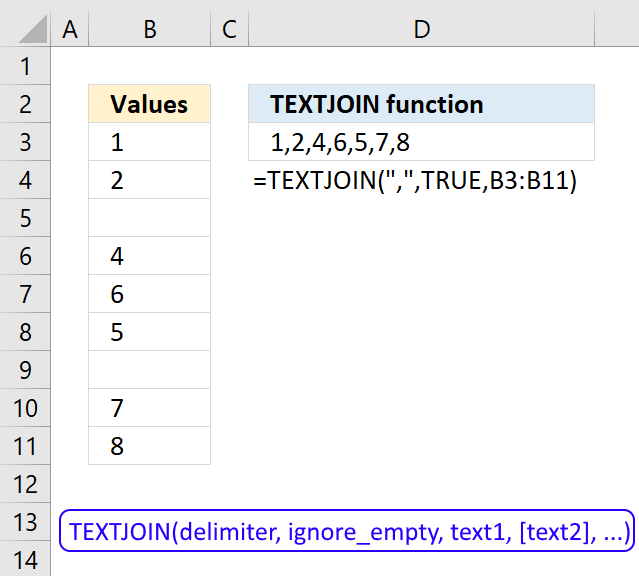The TEXTJOIN function is a relatively new function introduced in Excel 2019, it is like the CONCATENATE function on steroids or what the CONCATENATE function should have been from the beginning.

It allows you to combine text strings from multiple cell ranges and also use delimiting characters if you want. Not only that, you can also use it in array formulas.

## 1. TEXTJOIN Function Syntax

TEXTJOIN(delimiter, ignore_empty, text1, [text2], ...)

## 2. TEXTJOIN Function Arguments

 delimiter Required. The delimiting character or characters you want to use. ignore_empty Required. True lets you ignore empty cells in the third argument, False adds empty cells to the output. text1 Required. The cell range you want to concatenate. [text2] Optional. Up to 254 additional cell ranges.

The similar CONCATENATE function forced you to select each cell in order to join text strings, see picture below. If you had to combine many many cells this function was not an option, you had to use a custom vba function.

You can use the TEXTJOIN function with a cell range or multiple cell ranges, this may save you a lot of time if you are working with many cells.

## 3. How to join cell values without a delimiting character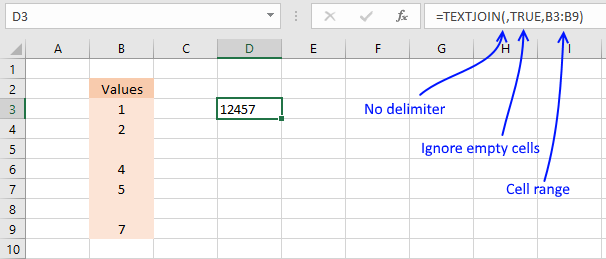The first argument in the TEXTJOIN function is the delimiter, the example above shows you the result in cell C4 if you use no delimiting characters.

The second argument lets you choose between TRUE or FALSE, if TRUE it will ignore empty cells. The third argument is the cell range.

The following article shows you how to add unique distinct values to a cell using the TEXTJOIN function:

Recommended articles

Concatenate unique distinct values
This article demonstrates Excel formulas that extract unique distinct values from a cell range and concatenates the values using any […]

## 4. How to concatenate cell values ignoring empty cells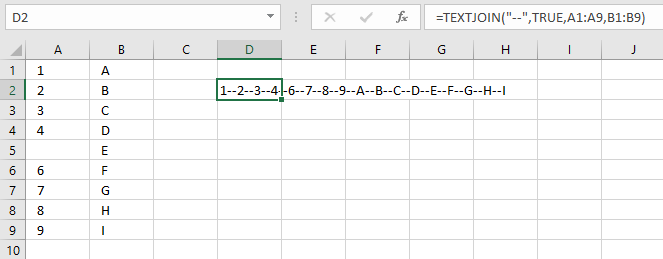This picture shows you the TEXTJOIN function combining multiple cell ranges with a delimiting text string. It also ignores the empty cell A5. You can use up to 252 cell ranges.

## 5. How to concatenate values based on a condition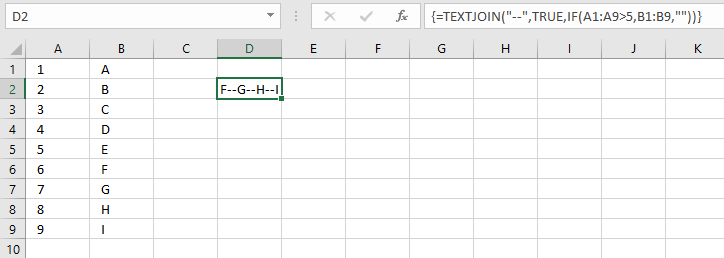It is possible to use the TEXTJOIN function in an array formula, here is a simple demonstration. You can see that the third argument contains:

IF(A1:A9>5,B1:B9,"")

This IF function checks if the numbers in cell range A1:A9 are above 5 and if they are the corresponding value in cell range B1:B9 is returned.

Numbers 6,7,8 and 9 are larger than 5 so corresponding characters F, G, H, and I are combined using the delimiting characters "--".

### 5.1 Explaining formula

#### Step 1 - Logical expression

The larger than character is a logical operator that lets you check if a number is larger than another number, the result is a boolean value TRUE or FALSE.

A1:A9>5

becomes

{1; 2; 3; 4; 5; 6; 7; 8; 9}>5

and returns

{FALSE; FALSE; FALSE; FALSE; FALSE; TRUE; TRUE; TRUE; TRUE}.

#### Step 2 - Evaluate IF function

The IF function returns one value if the logical test is TRUE and another value if the logical test is FALSE.

IF(logical_test, [value_if_true], [value_if_false])

IF(A1:A9>5,B1:B9,"")

becomes

IF({FALSE; FALSE; FALSE; FALSE; FALSE; TRUE; TRUE; TRUE; TRUE},{"A"; "B"; "C"; "D"; "E"; "F"; "G"; "H"; "I"},"")

and returns

{""; ""; ""; ""; ""; "F"; "G"; "H"; "I"}.

#### Step 3 - Join values

TEXTJOIN("--", TRUE, IF(A1:A9>5, B1:B9, ""))

becomes

TEXTJOIN("--", TRUE, {""; ""; ""; ""; ""; "F"; "G"; "H"; "I"})

and returns

"F--G--H--I".

This post demonstrates how to do a lookup and concatenate returning values:

Recommended articles

Lookup and return multiple values concatenated into one cell
This article demonstrates how to find a value in a column and concatenate corresponding values on the same row. The […]

## 6. TEXTJOIN function VBA for previous Excel versions

The following user defined function (udf) allows you to combine text strings just like the TEXTJOIN function.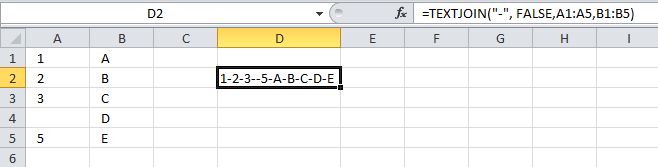Why would you want to use this udf? TEXTJOIN function is only available in Excel 365, previous excel versions are missing this function.

### 6.1 VBA code

```'Name User Defined Function, specify parameters and declare data types
Function TEXTJOIN(delimiter As String, ignore_empty As Boolean, ParamArray cell_ar() As Variant)

'For each statement, iterate through each cell range
For Each cellrng In cell_ar

'For each statement, iterate through each cell in cell range
For Each cell In cellrng

'If ... Then ... Else ... End If statement
'Check if parameter ignore_empty is equal to boolean value False
If ignore_empty = False Then

'Concatenate cell value and delimiter with variable result and save to result
result = result & cell & delimiter

'Go here if ignore_empty is not equal to False
Else

'Check if cell value is not equal to nothing
If cell <> "" Then

'Concatenate cell value and delimiter with variable result and save to result
result = result & cell & delimiter
End If
End If

'Continue with next cell value
Next cell

'Continue with next cell range
Next cellrng

'Remove last delimiter in variable result and return values to worksheet
TEXTJOIN = Left(result, Len(result) - Len(delimiter))
End Function
```

### 6.2 Where do I put this code?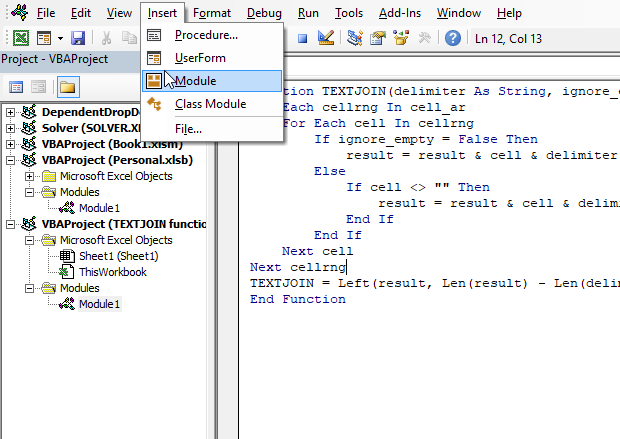1. Press Alt + F11 to open the Visual Basic Editor (VBE).
2. Press with left mouse button on "Insert" on the menu.
3. Press with left mouse button on "Module".
4. Copy code above.
5. Paste it to the code module.
6. Exit VB Editor.

### 6.3 How do I use it?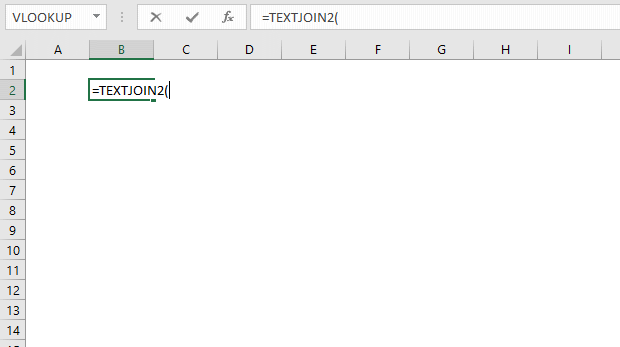1. Select a cell.
2. Type Textjoin(
3. Type your three arguments and ending parentheses.
4. Press Enter.

### 6.4 UDF arguments

delimiter - A text string, if you want nothing type ""

ignore_empty - If TRUE it ignores empty cells

text1, text2, ... - A cell range or multiple cell ranges. Use a comma to separate them.

### 6.5 UDF Syntax

TEXTJOIN(delimiter, ignore_empty, [text1, text2, ...])

## 7. TEXTJOIN function - how to add row delimiting characters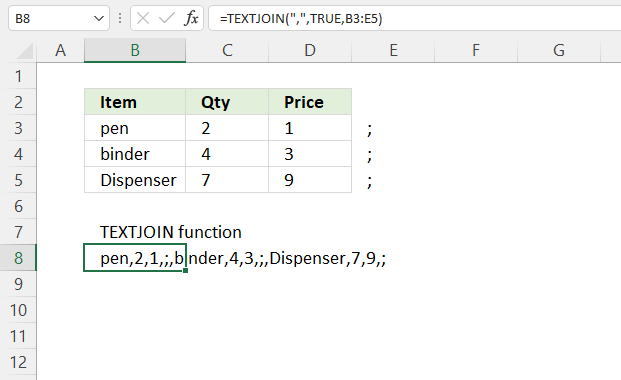The TEXTSPLIT function allows you to split a string using both column and row delimiting characters, however, the TEXTJOIN function lets you only use a column delimiting character.

There is a workaround to add row delimiting characters, add your row delimiting character after the last cell on each row, see the image above cell range E3:E5.

You can now use the TEXTSPLIT function to split the string to create a 2D array, 2D array is an array with both columns and rows.